# NPER In ExcelArticle byExcelMojo Team## What Is NPER Function In Excel?

The NPER function in Excel is an inbuilt Financial function. It determines the number of periods needed for a loan or an investment through regular payments at a constant interest rate. Users can use the function NPER in Excel to calculate the number of periods needed to reach their target while building a corporate fund.

For example, the below table contains the input values required to calculate the total payment periods needed to pay off a loan.

Suppose we need to show the number of periods in cell B10. Then using NPER in Excel cell B10 we can fetch the required output.

Thus, finding NPER in Excel in the above example shows that it will take 6.9 years to repay a loan of \$20,000 when we make a yearly payment of \$4,000 at the fixed interest rate of 9%.

###### Key Takeaways
• The function NPER in Excel calculates the number of periods for a loan or an investment to earn an amount via periodic payments at a fixed interest rate.
• The NPER() accepts three mandatory arguments, rate, pmt, and pv, and two optional arguments, fv, and type, as inputs.
• Suppose we omit pv or supply its value as 0. Then, we must provide the fv value to NPER().
• The argument type can be either 0 or 1. While the value 0 indicates the payments are due at the end of the period, the value 1 denotes the payments due at the beginning of the period.

### NPER() Excel Formula

The syntax of NPER in Excel is

where,

• rate: It is the interest rate per period.
• pmt: The payment made each period. And it includes principal and interest but no other fees and taxes.
• pv: It is the present value, or the lump-sum amount, a sequence of future payment values now.
• fv: The future value or the money balance we wish to achieve after making the last payment.
• type: It denotes when payments are due.

While the first three arguments in the function, NPER in Excel, are mandatory, the remaining arguments are optional.

Please Note: Typically, we will pay long-term loans in monthly installments and others quarterly or semi-annually. Thus, to find the number of periodic payments required to repay the loan, we can find the periodic rate by dividing the given annual interest rate by the number of periods per year.

Also, when using the function to calculate NPER in Excel, ensure we adhere to the following points to avoid potential errors.

• The argument rate can be a percentage or decimal value.
• If the argument pv is 0 or you ignore it, we must supply the argument fv.
• If we ignore the argument fv, the function considers it as 0.
• If we supply the argument type as 0 or ignore it, it denotes the payments are due at the period end. And a value of 1 indicates the payments to be due at the start of the period.
• All incoming and outgoing payments should be positive and negative numbers, respectively.
• If the future value is not attainable or the periodic interest rate and payments are inadequate, the function NPER in Excelthrows the #NUM! error.
• If the supplied arguments are non-numeric values, the NPER() will return the #VALUE! error.

### How To Use NPER Excel Function?

The steps to apply the function NPER in Excel are:

1. First, ensure all the payment values have the correct signs and that none of the supplied NPER() arguments are non-numeric.
2. Next, select the target cell and enter the function NPER in Excel.
3. Finally, press Enter to view the required number of payment periods.

Let us see the above steps to apply the function NPER in Excel with example.

Consider we want to invest \$15,000 and aspire to make \$400,000. Let the annual interest rate be 7%. And assume we will make an additional contribution of \$4,000 at each month end.

So, here is how we can determine the number of monthly investments we need to make to earn \$400,000 using NPER in Excel.

1. To begin with, select the target cell B11, enter the NPER(), and press Enter.

=NPER(C5/12,C6,C7,C8,C9)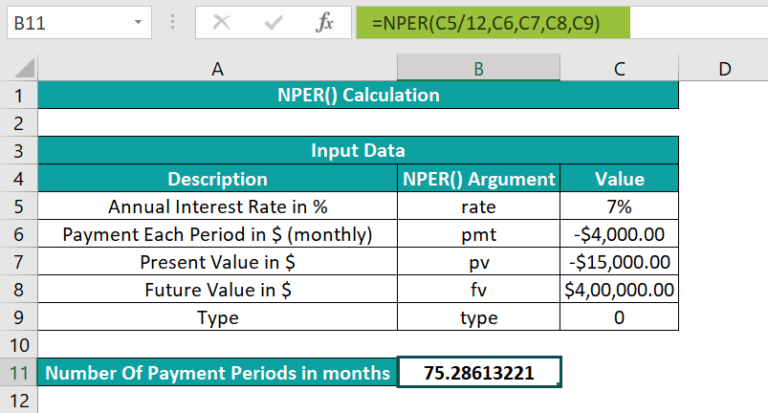Alternatively, we can apply the NPER() from the Formulas tab by clicking Formulas à Financial à NPER to open the Function Arguments window.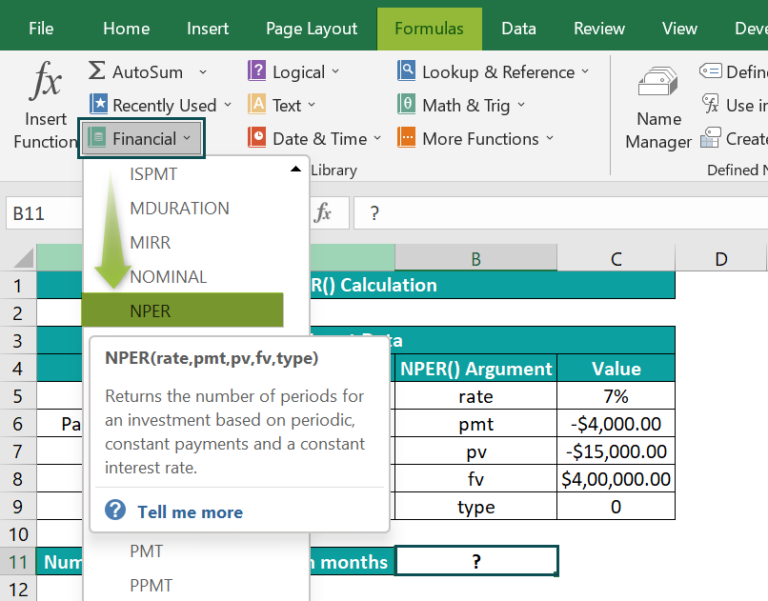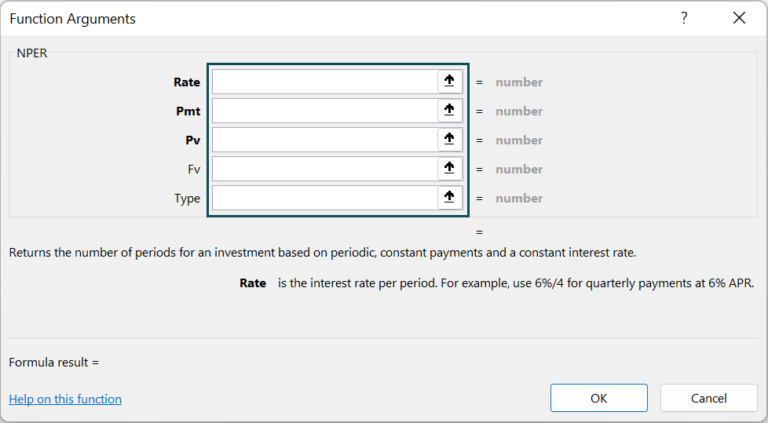Next, enter the NPER() argument values in the Function Arguments window.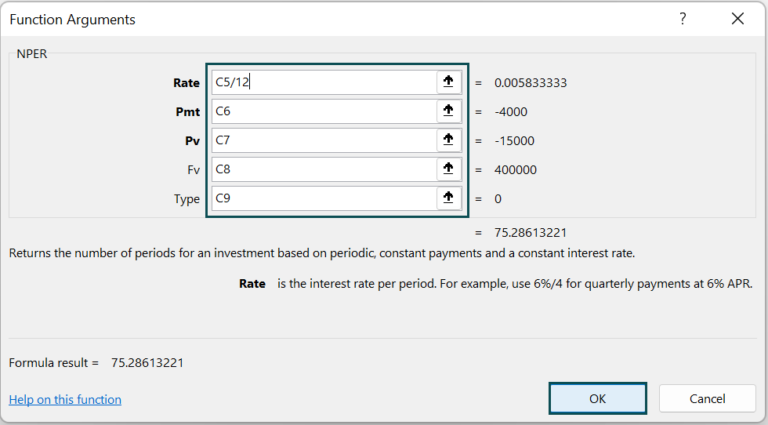Finally, when we click OK in the Function Arguments window, the NPER() will get executed in the target cell.

In this example, the installments are monthly. So, while finding NPER in Excel, we shall use the periodic rate as the annual interest rate/12. And as the monthly payments and present values are money outflows, they have a negative sign. Also, as the payments are due at the end of each period, we take the default value for the argument type, 0.

Thus, based on the above inputs, when we calculate NPER in Excel, we get the result of 75.28 months.

### Examples

The previous section explained the function NPER in Excel with example. Here we shall see a few more illustrations to best use the function.

#### Example #1

Consider we require a loan of \$20,000 at an annual interest rate of 5%. And we can pay \$1,000 at the beginning of each month to repay the loan.

Suppose we need to determine the number of payment periods to pay off the loan based on the given data and display the output in cell B10. Then, we can apply the function NPER in Excel in the target cell and get the required months count.

• Step 1: First, select the target cell B10, enter the following NPER(), and press Enter.

=NPER(C5/12,C6,C7,,1)

After we enter the rate, pmt, and pv values, enter two commas to show the argument fv omission. Then, we will see a drop-down list to select the argument type.

In the example, as the payment is at the start of each period, the argument type value is 1.

And once we close the parenthesis and press Enter, the NPER() gets executed.

We can also enter the function NPER in Excel as shown below:

=NPER(C5/12,C6,C7,,C8)

As the loan repayment will be monthly, we divide the annual interest rate by 12. And, thus based on the above inputs, the NPER() returns the number of payment periods to repay the \$20,000 loan as 20.83 months.

#### Example #2

We can create a universal formula for NPER in Excel to calculate the payment periods, whether for a mortgage or to earn an amount.

The below image shows a table with the required inputs and a legend to select the argument type and payment periods per year.

And suppose we require to show the number of payment periods in cell B12. Then we can apply the universal NPER in Excel formula in the target cell to achieve the data, whether to borrow or save an amount.

For example, we aim to invest an initial \$15,000 at an annual interest rate of 6%. And we make an additional contribution of \$1,000 at the start of each semi-annual period, with the target to reach \$60,000.

And suppose we need to calculate the number of semi-annual payment periods required to reach \$60,000 and display the output in cell B12. Then the universal NPER() we can apply in the target cell is:

• Step 1: To begin with, select the target cell B12, enter the following NPER(), and press Enter.

=NPER(C5/C10,C6,C7,C8,C9)

We can refer to the legend for the argument type and the Payment Periods Per Year value. In this example, the payment is at the start of each semi-annual period. And thus, the argument type value is 1, and the Payment Periods Per Year value is 2.

So, based on the payment periods per year referred from the legend table, the first argument in the NPER() in cell B12, rate, gets divided by the value in cell C10. And we need to enter the remaining argument values.

Hence, with the given inputs, the NPER() formula returns 14.56 semi-annuals as the number of periods to attain the target of \$60,000.

#### Example #3

This example shows how to use NPER with the ROUND excel function to achieve the number of periods as a whole number.

Suppose we wish to avail a loan of \$30,000 at an annual interest rate of 10%. And we can make yearly payments of \$5,000, with the payments being at the end of each period.

And we must determine the number of periods to repay the loan of \$30,000 as a whole number in cell B10. Then, here is how we can apply the function NPER in Excel with the ROUND() in the target cell and achieve the required data.

• Step 1: First, select the target cell B10, enter the below formula, and press Enter.

=ROUND(NPER(B5,B6,B7),0)

The NPER() takes the input data as the first three mandatory arguments. And as payment is at the end of each period, the function takes the argument type default value, 0.

So, based on the given inputs, the NPER() returns the required number of periods to repay the loan of \$30,000 as 9.61377 years. And finally, the ROUND() rounds off the value 9.61377 to 0 decimal places, thus returning the number of periods as a whole value, 10 years.

### Important Things to Note

• We need to ensure to provide the rate according to payment periods per year, semi-annually, quarterly, or monthly.
• We must check whether the money inflow and outflow values are positive and negative, respectively.
• In case the future value is not viable or periodic interest rate and payments are insufficient, then, the function NPER in Excel will return the #NUM! error.
• The NPER in excel function does not take arguments which are not non-numeric. Otherwise, the function will return #VALUE! error.

1. Where is the NPER function in Excel?

The NPER function in Excel is in the Formulas tab. We may click FormulasFinancialNPER to access it.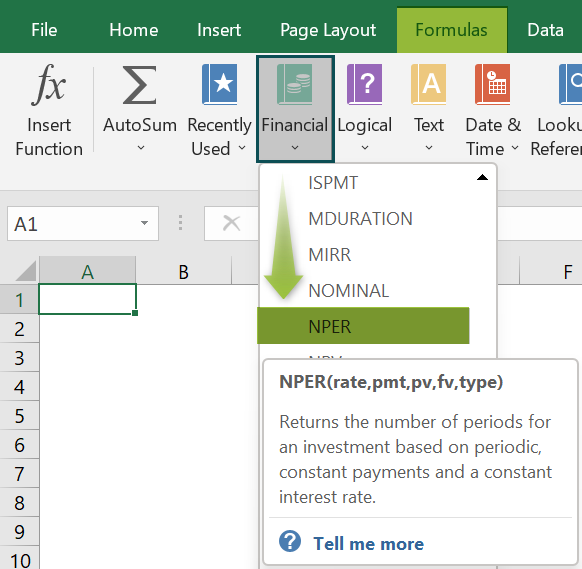2. How to apply the NPER function in Excel VBA?

We can apply the NPER function in Excel VBA using the method:

Nper(rate,pmt,pv,fv,type)

The arguments in the above function have the same interpretation as explained in the above article.

Let us see an illustration of applying the NPER function in Excel VBA.

Consider we need to avail a loan of \$25,000 at an annual interest rate of 4.5%. And we can make quarterly payments of \$6,000, with the payments made at the end of each period.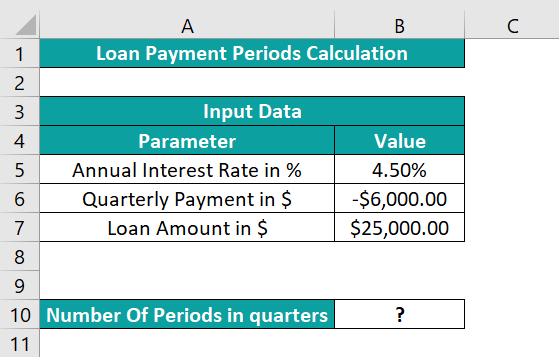And the requirement is to display the output in cell B10. So then, here is how we can use the NPER function in Excel VBA to get the required result in the target cell.

• Step 1: To begin with, press the keys Alt + F11 with the worksheet with the above table open, to open the VBA Editor.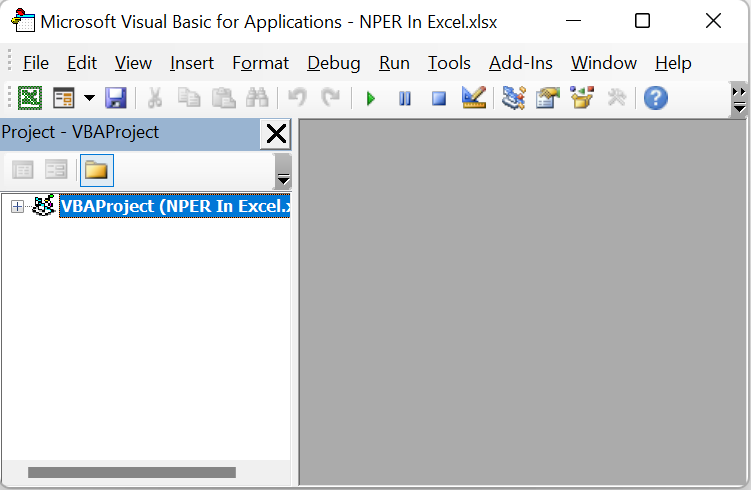• Step 2: Next, select the required VBAProject and click InsertModule to open a new module window, Module1.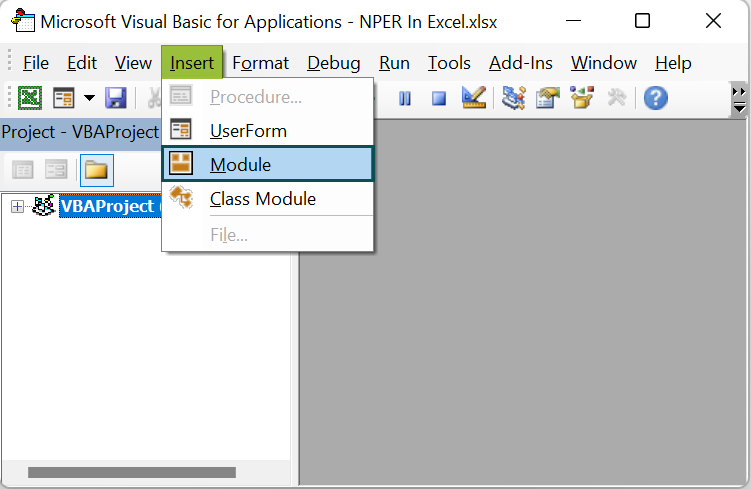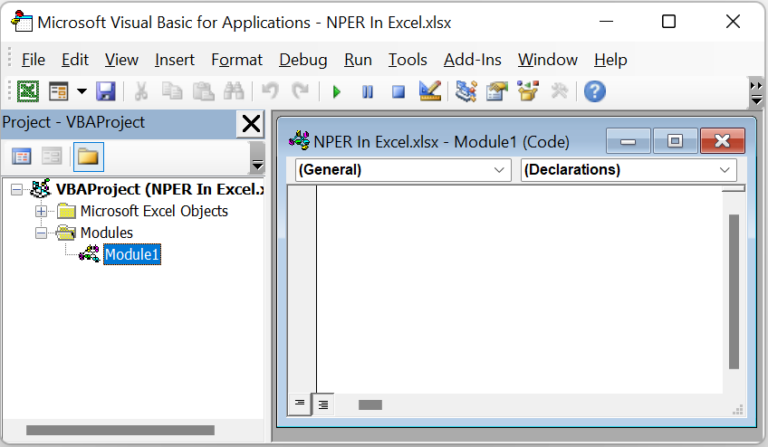• Step 3: Now, type the VBA code in the Module1 window to apply the NPER() in the target cell.

Sub NPER_fn()
Range(“B10”).Value = NPer(Range(“B5”).Value / 4, Range(“B6”).Value, Range(“B7”).Value)
End Sub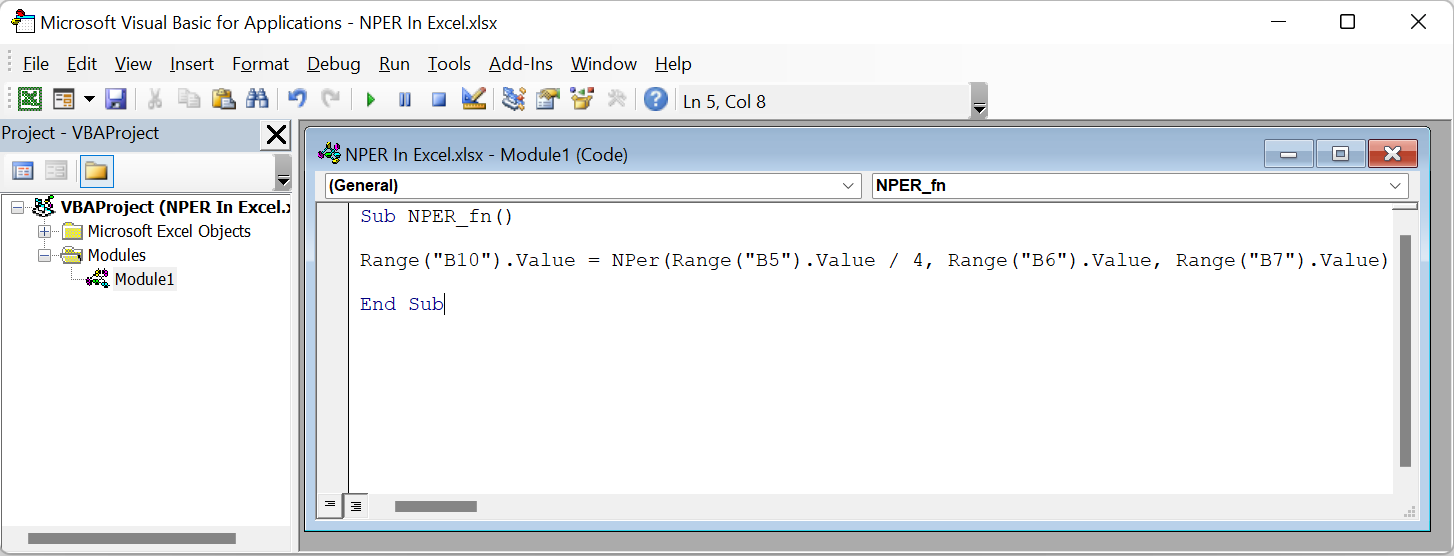• Step 4: Then, click the Run Sub/UserForm icon to run the commands in the Module1 window.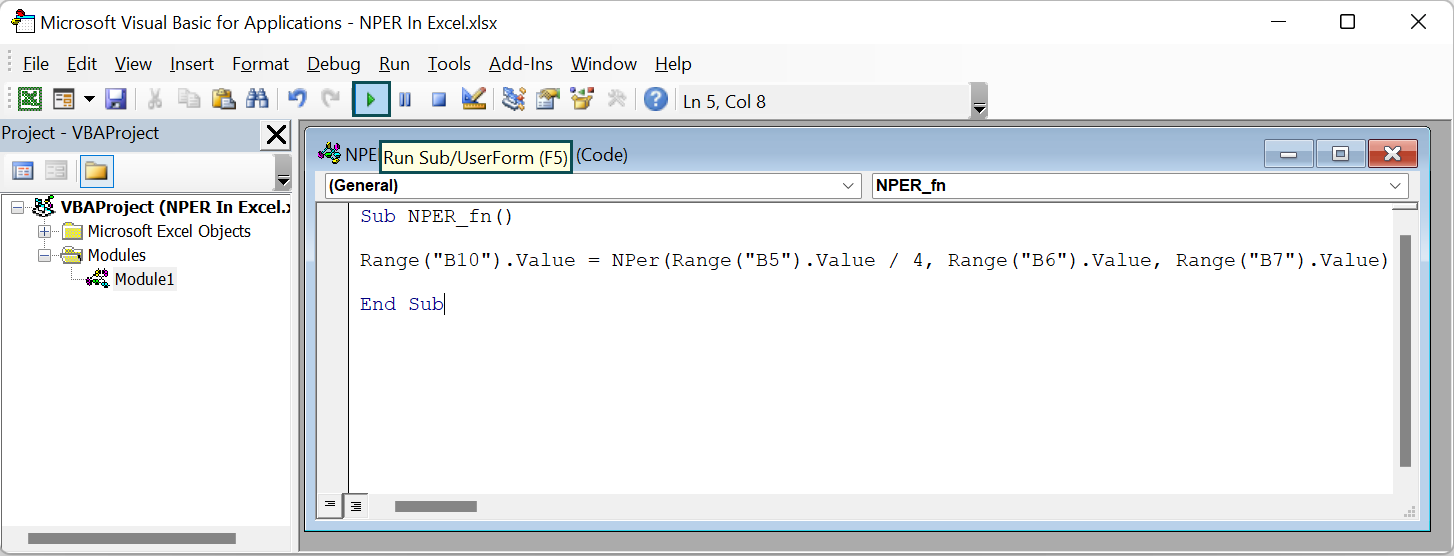If we open the worksheet containing the source table, we will find the NPER() executed and the required output in the target cell B10.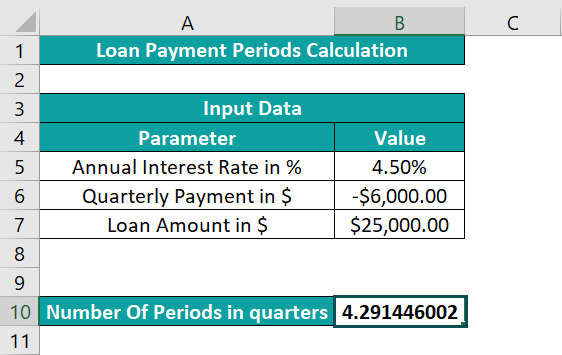In the above example, the payments are quarterly. Hence, we divide the annual interest rate by 4 in the NPER(). And with the rest of the mandatory arguments provided, the function determines the number of periods as 4.29.

3. Why is the Excel NPER not working?

The Excel NPER is not working, perhaps due to the following reasons:

We did not provide the argument rate corresponding to the required payment periods per year.
We did not provide the correct signs for the cash flow values.
The provided future value is not achievable, or the periodic interest rate and payments are inadequate.
The supplied arguments are non-numeric.